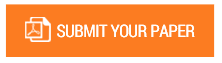Exclusive Sum Labeling on Gear Graphs

Authors : Chrisaria Palungan; Nurdin Hinding; Muhammad Zakir

Volume/Issue : Volume 7 - 2022, Issue 12 - December

Scribd : https://bit.ly/3ZEa3xS

DOI : https://doi.org/10.5281/zenodo.7537942

A graph with an injective mapping from vertex G to positive integers S such that two vertexes of G adjacent if and only if sum of labels can be an element of S. The aim of this research is to constructing exclusive sum labeling on gear graphs and obtaining isolated vertex sums for exclusive sum labeling on gear graphs. The data will be categorized in exclusive sum labeling on gear graph by Leonhard Euler. There are systematics in proving the theorem will be presented in the following steps: Defining labels for each vertex in a graph with adjacent sum of label is the label of isolated vertex. Proving the isolated vertex has obtained an optimal. This research conducted to find the exclusive sum labeling on gear graph by producing the optimal in exclusive sum labeling. In an exclusive addition of the integers of S which is the sum of two other integers of S can be labeling a set of isolated vertex which related to G graph. This article can show the construction of exclusive sum labeling on gear graphs.

Keywords : Gear Graph, Sum Labeling, Exclusive Sum Labeling

#### CALL FOR PAPERS

Paper Submission Last Date
31 - March - 2023